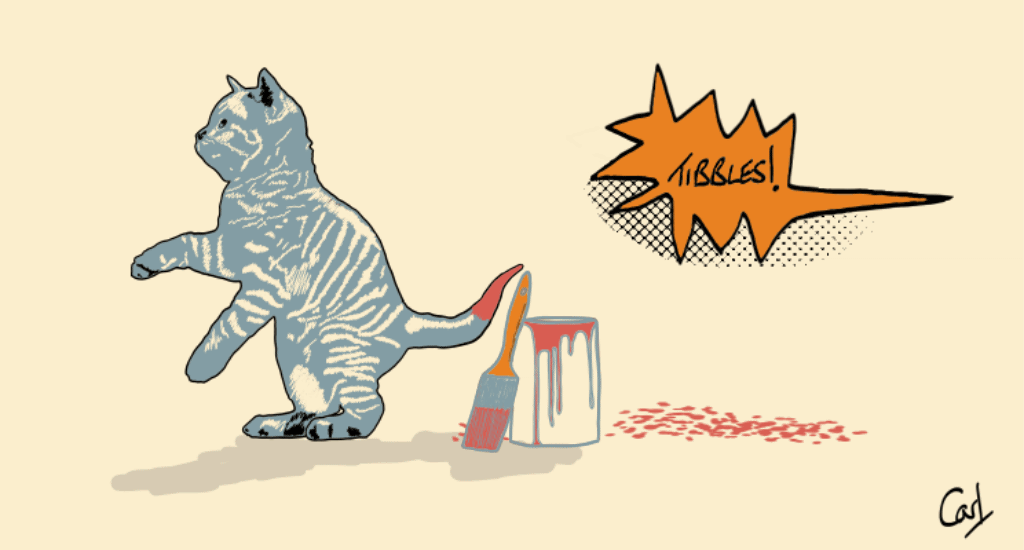If you’re a cat, go find the nearest open pot of paint. No need to read further.

But if you’re a data scientist looking to paint the tail of a density plot, what to do?

There are techniques for painting a region under a curve. But the experimental ggfx package offers an interesting alternative solution based on the blending modes familiar to users of Photoshop.

library(tidyverse)
library(scales)
library(ggfx)
library(patchwork)
library(wesanderson)
library(clock)
library(tidyquant)

theme_set(theme_bw())

(cols <- wes_palette("Royal1"))The advantage here is that the tail-painting aesthetic needs no information about the shape of the curve; only the limits on the x-axis.

The left plot shows the raw components without blending. The right plot is only retaining the red where there is a layer below.

p0 <- tibble(outcome = rnorm(10000, 20, 2)) |>
ggplot(aes(outcome)) +
scale_y_continuous(labels = label_percent())

p1 <- p0 +
geom_density(adjust = 2, fill = cols) +
annotate("rect",
xmin = 15, xmax = 18, ymin = -Inf, ymax = Inf,
fill = cols
) +
labs(title = "Without Blending", y = "Density")

p2 <- p0 +
as_reference(geom_density(adjust = 2, fill = cols), id = "density") +
with_blend(annotate("rect",
xmin = 15, xmax = 18, ymin = -Inf, ymax = Inf,
fill = cols
), bg_layer = "density", blend_type = "atop") +
labs(title = "With Blending", y = NULL)

p1 + p2Of course the red box could also be layered behind a density curve with alpha applied so it shows through. But if the preference is tail-only colouring, it’s a neat solution.

Blending is actually a handy solution for any awkward shape. The same technique is used here with a time series ribbon summarising the median, lower and upper quartiles of a set of closing stock prices.

tickrs <- c("AAPL", "NFLX", "TSLA", "ADBE", "FB", "GOOG", "MSFT")

p0 <- tq_get(tickrs, get = "stock.prices", from = "2022-01-01") |>
group_by(date) |>
summarise(
close = quantile(close, c(0.25, 0.5, 0.75)),
quantile = c("lower", "median", "upper") |> factor()
) |>
ungroup() |>
pivot_wider(names_from = quantile, values_from = close) |>
ggplot(aes(date, median)) +
annotate("text",
x = as.Date("2022-03-16"), y = 100,
label = "Helpful\nAnnotation", colour = "black"
) +
scale_y_continuous(limits = c(0, NA)) +
labs(x = NULL)

p1 <- p0 +
geom_ribbon(aes(ymin = lower, ymax = upper), fill = cols) +
geom_line(colour = cols) +
annotate("rect",
xmin = as.Date("2022-03-01"), xmax = as.Date("2022-03-31"),
ymin = -Inf, ymax = Inf, fill = cols, colour = "black", linetype = "dashed"
) +
labs(title = "Without Blending", y = "Closing Price")

p2 <- p0 +
as_reference(geom_ribbon(aes(ymin = lower, ymax = upper), fill = cols), id = "ribbon") +
with_blend(
annotate(
"rect",
xmin = as.Date("2022-03-01"), xmax = as.Date("2022-03-31"),
ymin = -Inf, ymax = Inf, fill = cols, colour = "black", linetype = "dashed"
),
bg_layer = "ribbon", blend_type = "atop"
) +
geom_line(colour = cols) +
labs(title = "With Blending", y = NULL)

p1 + p2 +
plot_annotation(title = "Median Price Bounded by Upper & Lower Quartiles")It would be interesting to hear about other uses of ggfx beyond the purely artistic.

Posted:
April 26, 2022
Updated:
May 7, 2022
Length:
3 minute read, 506 words
Categories:
R
Tags:
special effects